Question

# If X and Y have a bivariate normal distribution with parameters mean1,mean2, variance1, variance2and P show...

If X and Y have a bivariate normal distribution with parameters mean1,mean2, variance1, variance2and P show that Z = aX + bY + c is N(a.mean1 + b.mean2 + c, variance1.variance2 + 2abp.variance1.variance2 + b^2.variance2, where a, b, and c are constants. Hint: Use the m.g.f. M(t1 t2) of X and Y to find the m.g.f. of Z.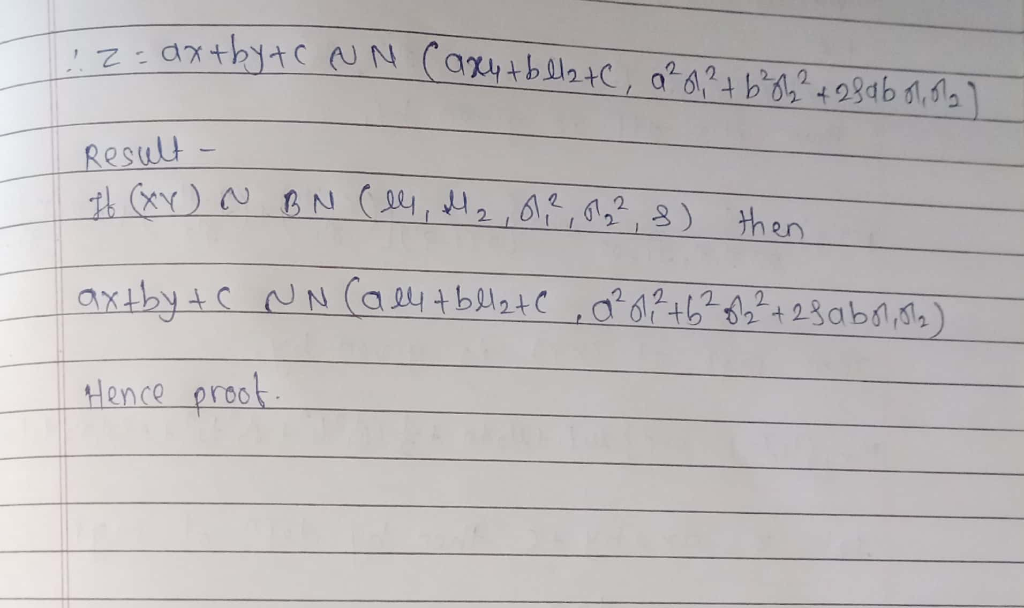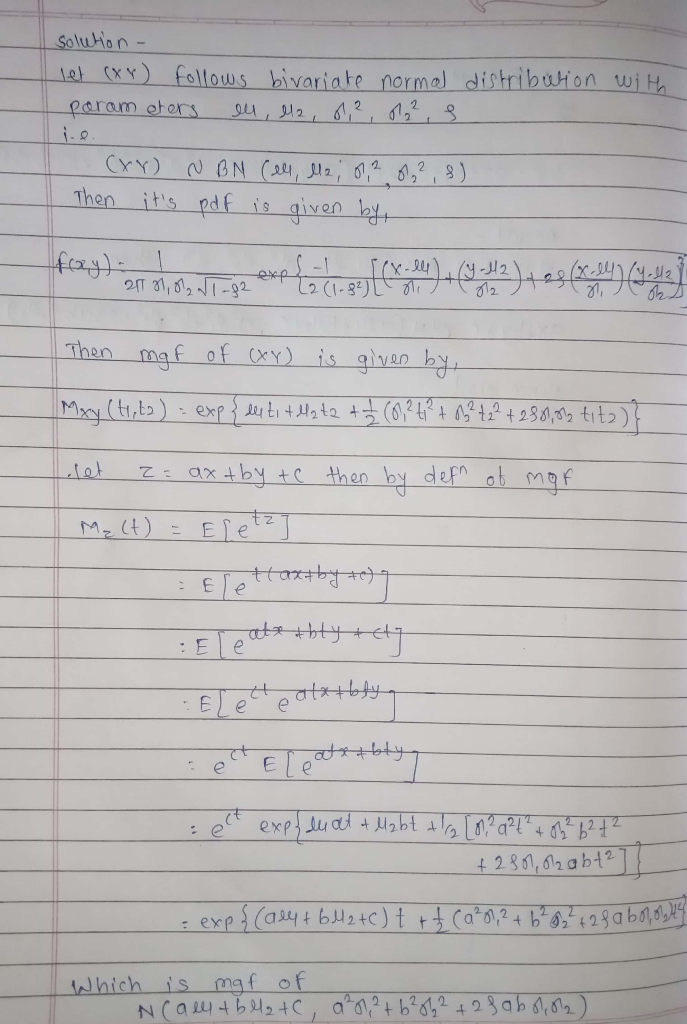#### Earn Coins

Coins can be redeemed for fabulous gifts.

Similar Homework Help Questions
• ### Let X and Y have a bivariate normal distribution with parameters μX = 10, σ2 X = 9, μY = 15, σ2 Y = 16, and ρ = 0. Find (a) P(13.6 < Y < 17.2). (b) E(Y | x). (c) Var(Y | x). (d) P(13.6 < Y &l...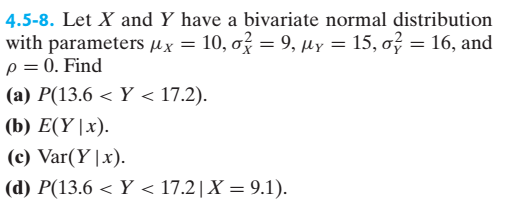Let X and Y have a bivariate normal distribution with parameters μX = 10, σ2 X = 9, μY = 15, σ2 Y = 16, and ρ = 0. Find (a) P(13.6 < Y < 17.2). (b) E(Y | x). (c) Var(Y | x). (d) P(13.6 < Y < 17.2 | X = 9.1).   4.5-8. Let X and Y have a bivariate normal distribution with parameters Ax-10, σ(-9, Ily-15, σǐ_ 16, and ρ O. Find (a) P(13.6< Y < 17.2)...

• ### 17. Suppose that (X,Y) has a bivariate normal distribu- zion with parameters diy, x, 0y.p. io...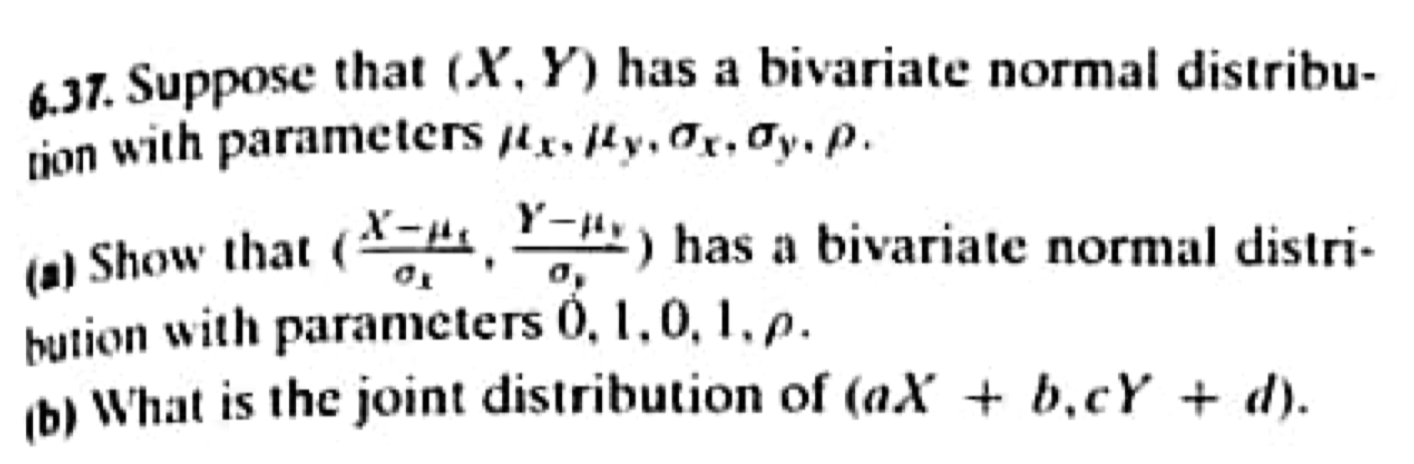17. Suppose that (X,Y) has a bivariate normal distribu- zion with parameters diy, x, 0y.p. io show that (2 , 4") has a bivariate normal distri- bution with parameters 0, 1,0.1.p. b) What is the joint distribution of (aX + b,cY + d).

• ### 3. Let X and Y have a bivariate normal distribution with parameters x -3 , μΥ...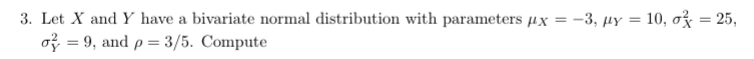3. Let X and Y have a bivariate normal distribution with parameters x -3 , μΥ 10, σ 25, 9, and ρ 3/5. Compute (c) P(7<Y < 16). (d) P(7 < Y < 161X = 2).

• ### please help me 6. Suppose X, Y have a bivariate normal distribution with marginal dis- tribution...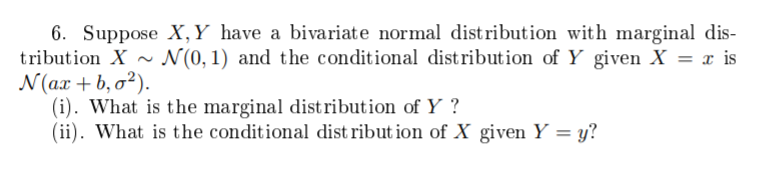please help me 6. Suppose X, Y have a bivariate normal distribution with marginal dis- tribution X ~ N(0,1) and the conditional distribution of Y given X-x is N(ax + b,a?). (i). What is the marginal distribution of Y? (ii). What is the conditional dist ribut ion of X given Y-y?

• ### bos on 559 2. Random variable X and Y have a bivariate normal distribution. The conditional...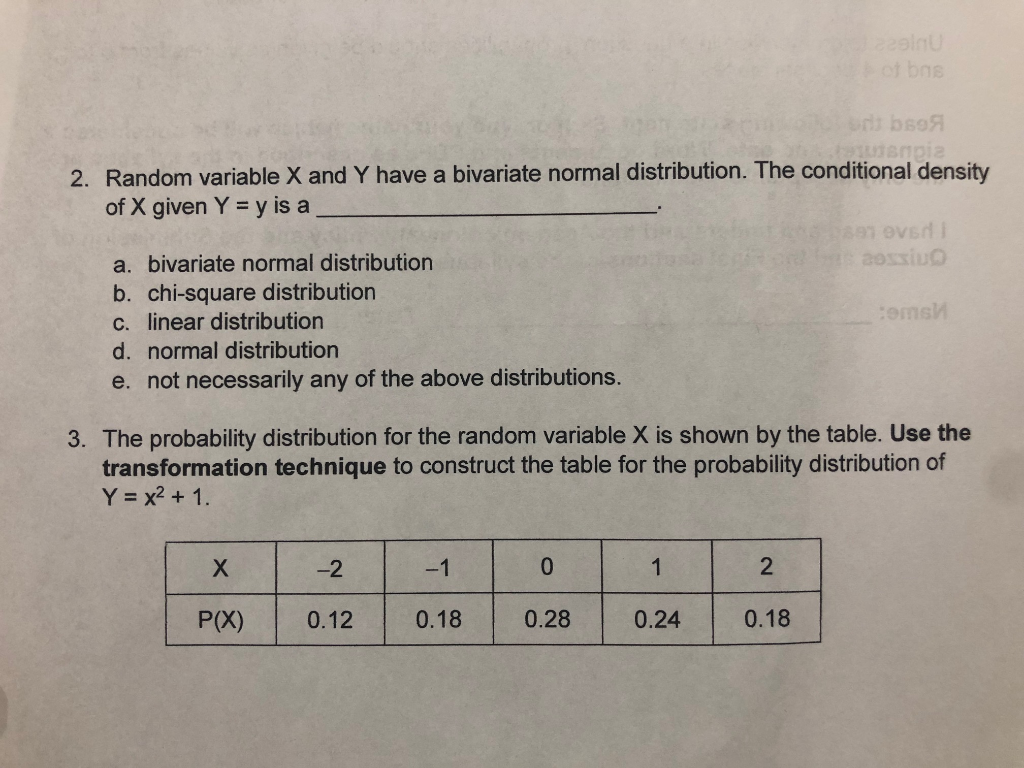bos on 559 2. Random variable X and Y have a bivariate normal distribution. The conditional density of X given Y = y is a OVH a. bivariate normal distribution Bossiu b. chi-square distribution c. linear distribution oms d. normal distribution e. not necessarily any of the above distributions. 3. The probability distribution for the random variable X is shown by the table. Use the transformation technique to construct the table for the probability distribution of Y = x2 +...

• ### 6. Suppose that X and Y have a bivariate normal distribution with px 1 and y- (a) Order the follo...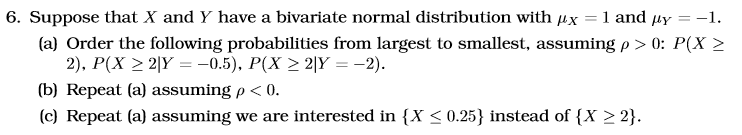6. Suppose that X and Y have a bivariate normal distribution with px 1 and y- (a) Order the following probabilities from largest to smallest, assuming p >0: P(X 2 (b) Repeat (a) assuming p < 0. (c) Repeat (a) assuming we are interested in (X 0.25) instead of (x 2 2). 6. Suppose that X and Y have a bivariate normal distribution with px 1 and y- (a) Order the following probabilities from largest to smallest, assuming p >0:...

• ### Suppose that (W,Z) have a bivariate normal distribution, that W ~N(0,1), and that the conditional distribution...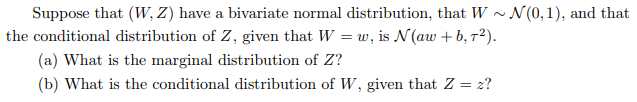Suppose that (W,Z) have a bivariate normal distribution, that W ~N(0,1), and that the conditional distribution of Z, given that Ww, is N(aw b,T2). (a) What is the marginal distribution of Z? (b) What is the conditional distribution of W, given that Z2?

• ### 4. Let (X,Y) be a bivariate normal random vector with distribution N(u, 2) where -=[ 5...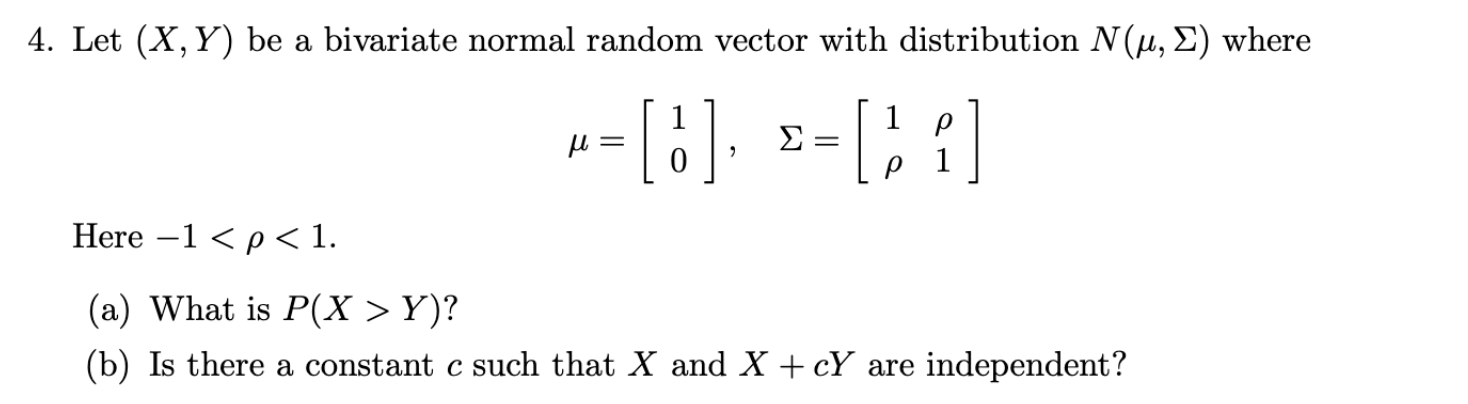4. Let (X,Y) be a bivariate normal random vector with distribution N(u, 2) where -=[ 5 ], =  Here -1 <p<1. (a) What is P(X > Y)? (b) Is there a constant c such that X and X +cY are independent?

• ### 6. Suppose that (W, Z) have a bivariate normal distribution, that W~N(0, 1), and that the...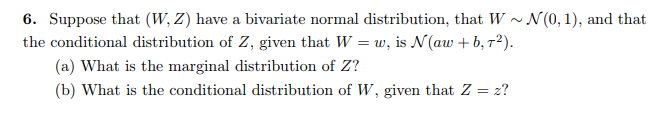6. Suppose that (W, Z) have a bivariate normal distribution, that W~N(0, 1), and that the conditional distribution of Z, given that W-w, is N(aw b, T2). (a) What is the marginal distribution of Z? b) What is the conditional distribution of W, given that Z-2?

• ### Suppose X and Y have a bivariate normal distribution with ox = 0.04, oy = 0.08,...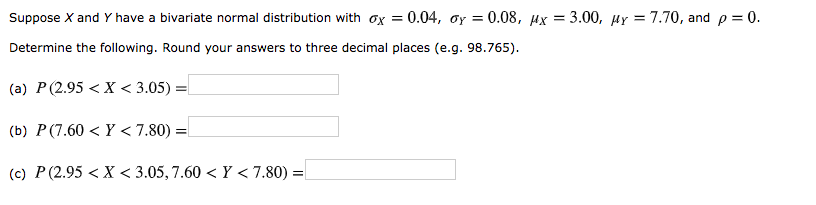Suppose X and Y have a bivariate normal distribution with ox = 0.04, oy = 0.08, Mx = 3.00, My = 7.70, and p = 0. Determine the following. Round your answers to three decimal places (e.g. 98.765). (a) P (2.95< X < 3.05) = (b) P (7.60 <Y < 7.80) = (c) P (2.95 < X <3.05,7.60 <Y < 7.80) =